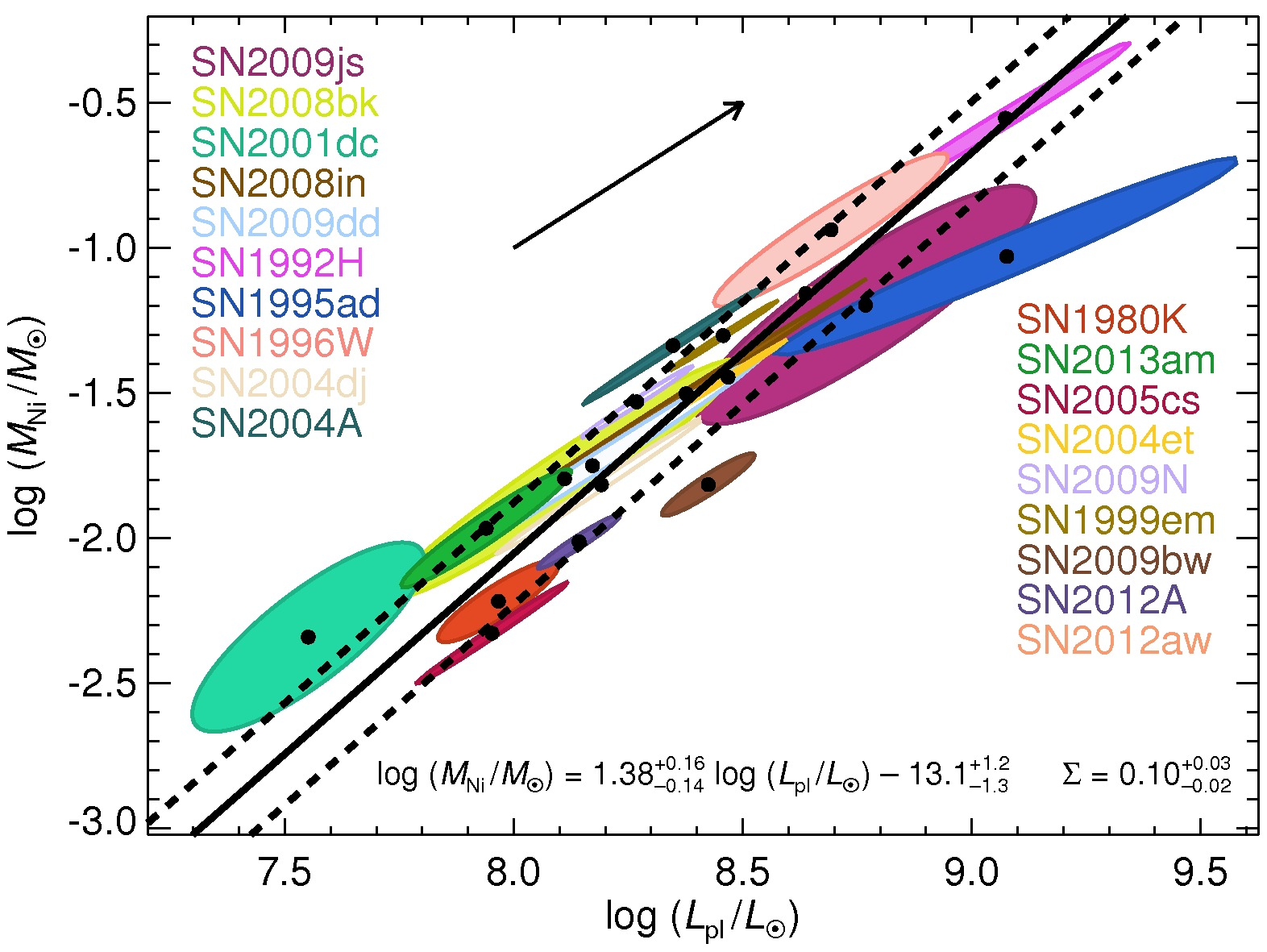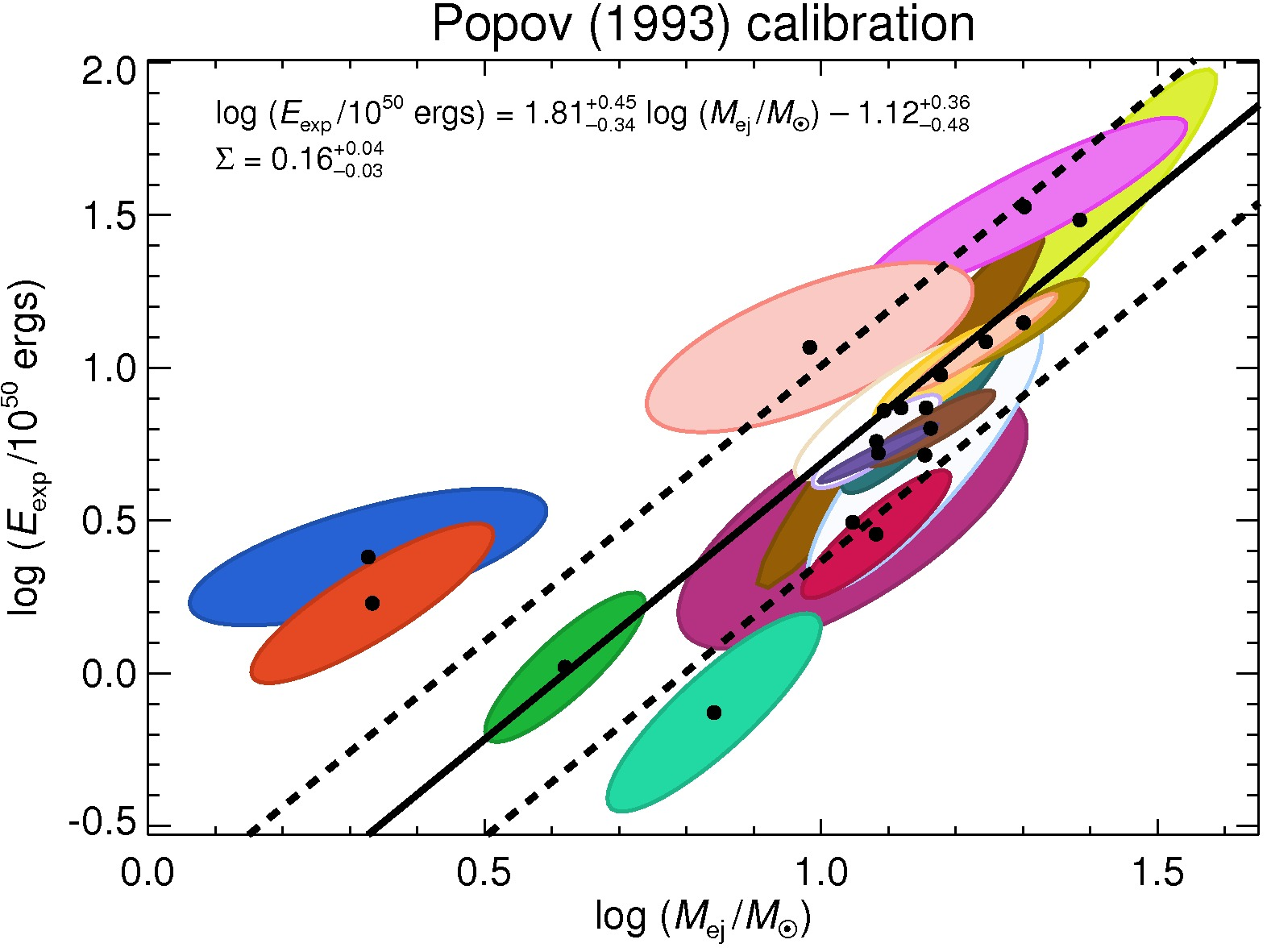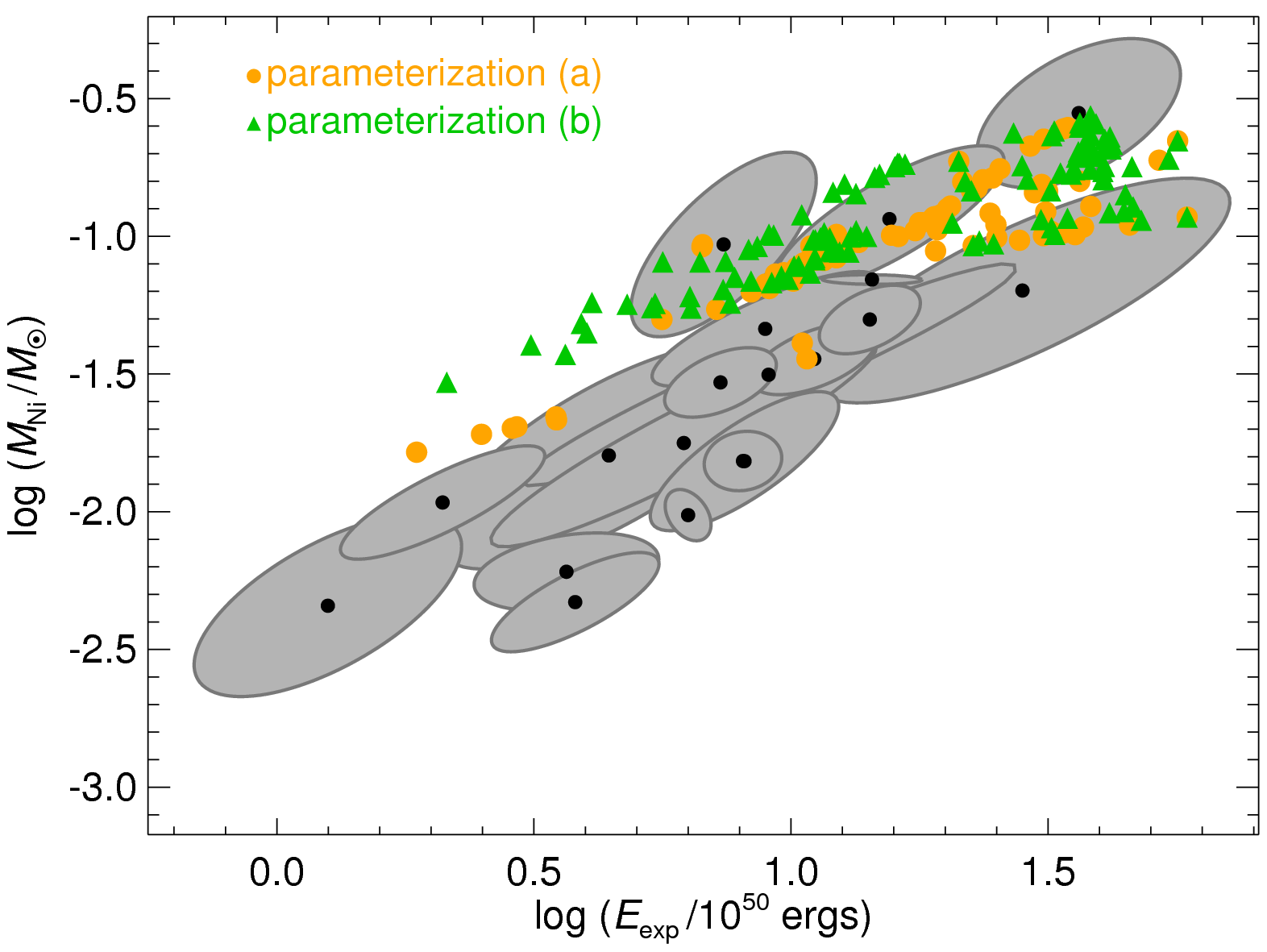## Physical parameters of Type II-Plateau Supernovae from Pejcha & Prieto (2015, ApJ, 806, 225)Comparison of observational results from Pejcha & Prieto (2015, ApJ, 806, 225) shown with grey ellipses with the predictions of the neutrino mechanism from Pejcha & Thompson (2015, ApJ, 801, 90) shown with orange and green symbols.

Here we give the plateau luminosities, nickel masses, explosion energies, and progenitor radii obtained using scaling relations from light curves fitted with the method of Pejcha & Prieto (2015, ApJ, 799, 215).

Litvinova & Nadezhin (1985) calibration of the scaling relations

Popov (1993) calibration of the scaling relations

Identification of supernova names

The column structure of the first two files is:

1. SN identification number (see the third file for correspondence to the common SN name)
2. Explosion epoch relative to the first datapoint in days (more negative number means less coverage of the initial part of the light curve)
3. Epoch of the last datapoint (useful to know when measuring late quantities like nickel mass)
4. log10 MNi - nickel mass in solar masses (=q0)
5. log10 Lbol - bolometric plateau luminosity at 50 days after explosion in solar luminosities (=q1)
6. log10 Eexp - explosion energy in 1050 ergs (=q2)
7. log10 Mej - ejecta mass in solar masses (=q3)
8. log10 R - progenitor radius in solar radii (=q4)
The subsequent columns are elements of the covariance matrix printed with a statement like
for (int i=0;i<5;i++) for (int j=0;j<5;j++) printf, Covar(qi, qj)

Sample IDL code to plot confidence ellipsoids like in our paper. covar is an array of covariance matrix coefficients obtained by reading the files above:

```plot1 = 2   ; variables to plot, here M_Ni vs E_exp
plot2 = 0

cov = reform(covar, 5,5)
my_cov[0,0] = cov[plot1,plot1]
my_cov[0,1] = cov[plot1,plot2]
my_cov[1,0] = cov[plot2,plot1]
my_cov[1,1] = cov[plot2,plot2]

invc = invert(my_cov,/double)
r2 = 2.30/(invc[0,0]*cp^2 + 2.0*invc[0,1]*cp*sp + invc[1,1]*sp^2)	;; factor 2.30 is related to number of variables and confidence level and is explained in Numerical Recipes
r = sqrt(r2)
x = r*cp	;; these are x and y coordinates of the 1-sigma confidence elipsoid relative to the datapoint
y = r*sp
```